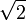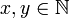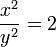# Square root of two

The square root of two, denoted$\sqrt{2}$, is the positive number whose square equals 2. It is approximately 1.4142135623730950488016887242097. It provides a typical example of an irrational number.

##  In Right Triangles

The square root of two plays an important role in right triangles in that a unit right triangle (where both legs are equal to 1), has a hypotenuse of$\sqrt{2}$. Thus,$\sin\left(\frac{\pi}{4}\right) = \cos\left(\frac{\pi}{4}\right)=\frac{1}{\sqrt{2}}=\frac{\sqrt{2}}{2}$.

##  Proof of Irrationality

There exists a simple proof by contradiction showing that$\sqrt{2}$ is irrational. This proof is often attributed to Pythagoras. It is an example of a reductio ad absurdum type of proof:

Suppose$\sqrt{2}$ is rational. Then there must exist two numbers,$x, y \in \mathbb{N}$, such that$\frac{x}{y} = \sqrt{2}$ and x and y represent the smallest such integers (i.e., they are mutually prime).

Therefore,$\frac{x^2}{y^2} = 2$ and$x^2 = 2 \times y^2$,

Thus, x2 represents an even number; therefore x must also be even. This means that there is an integer k such that$x = 2 \times k$. Inserting it back into our previous equation, we find that$(2 \times k)^2 = 2 \times y^2$

Through simplification, we find that$4 \times k^2 = 2 \times y^2$, and then that,$2 \times k^2 = y^2$,

Since k is an integer, y2 and therefore also y must also be even. However, if x and y are both even, they share a common factor of 2, making them not mutually prime. And that is a contradiction, so the assumption must be false, and$\sqrt{2}$ must not be rational.Some content on this page may previously have appeared on Citizendium.